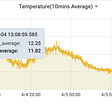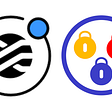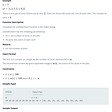# Dynamic Programming, you may have seen it before (ha ha)

Apart from being an acceptable way to get rushes of serotonin, dynamic programming is a way to cut down on time complexity.

So what is Dynamic Programming? Dynamic Programming is a practice where you break up a problem that is dependent on smaller problems and then optimize the time complexity of those problems to improve the time complexity of the overall problem.

Time complexity refers to the amount of time the program needs to run. There is a range of terms for the varying time complexities. The best one and shortest is linear time, which has one iteration per input. To be clear, if you have an array (a list) and you want to iterate through each one to console log them, that would be linear time.

`const nums = [1,2,3,4];    nums.map(num => {       console.log(num);    });`

Let's use a more complicated example, the infamous Fibonacci sequence. Dynamic programming varies in the amount it crushes downtime complexity depending on the original complexity. As such, I recommend writing a brute force solution first and then work on incorporating dynamic programming practices. The Fibonacci sequence is a list of numbers that follows a pattern where the previous and number previous to that one add together to equal the next one.

`//fibonacci sequence    const fibonacci = [1,1,2,3,5,8,13,21,34,55,89]; //goes on forever`

The general formula for determining the time complexity when incorporating dynamic programming is the number of unique calculations * time taken per calculation.

For the Fibonacci sequence, the brute force solution for finding the number at an nth place in the Fibonacci sequence goes something like this:

`const fibonacci = (n) => {    if (n <= 2) {        return 1;    }    return fibonacci(n - 1) + fibonacci(n - 2);};`

This implementation of finding the nth term in the Fibonacci sequence is done with recursion and has a time complexity of 2^n or exponential time.

This can be cut down through the implementation of dynamic programming and then you’ll hopefully get you fix of serotonin.

# Implementation:

## Memoization

One way to incorporate dynamic programming is through memoization. Memoization is where you store calculations that you plan on repeating so that you don’t have to recalculate them again later. This cuts down the time our program takes to run because it takes less time to get our stored values than it does to recalculate.

So, you’ll notice that in the Fibonacci example, we are repeating the same calculations when we call it with a value over 3. Here’s an example of the function call with the number 7:

In the graph above, the right side of the graph can be seen as a part of the left one. This means that we are recalculating this part all over again. Sure it may not take too long since our computer can handle it, but when the number becomes exceedingly high, the program will drastically slow down. So, how do we incorporate dynamic programming into the previous solution?

Since we want to make our program as fast as possible, we should have a way of storing and grabbing values from that storage in the fastest way possible. In javascript, we’d use an object since they store using key-value pairs that can be accessed in linear time through the key.

`const fibonacci = (n, memo = {}) => {    if(memo[n]){        return memo[n];    }    if (n <= 2) {        return 1;    }    return memo[n] = fibonacci(n - 1, memo) + fibonacci(n - 2,memo);};`

In the code above, there is a new parameter called memo, short for memoization. This object will function as storage that can be accessed in linear time using the nth term as the key. This causes the calculations to be cut down since we don’t have to recalculate any values that have already been calculated and stored in memo.

To recap, you use memorization to remember repeated calculations, make your code run faster, drown in serotonin, and make the world a better place.

## Tabulation

Some of you may still be craving that need for speed though. That’s understandable since we’re still recursing with memoization, so let’s try out tabulation.

Unlike memoization, tabulation involves tackling the problem from the base to the top. For instance, in memoization we begin at the nth term and work our way down to the first or second terms that then populate every term up till the nth term. When using tabulation, we start by looking at the first term and building our way up to the nth term from there.

Since we know how the Fibonacci sequence starts off as `0,1`, we can make an array off that and consistently push the new values into it and pull out the nth value using the index. Also, for those of you wondering, I start off the array at 0 because the Fibonacci sequence technically starts off at zero but it's unnecessary to include in the sum since anything plus zero is still zero.

Anyway, here’s the code for our tabulation:

`const fibonacci = (n) => {    const tab = [0,1];    for (let i = 2; i <= n; i++) {        tab[i] = tab[i - 1] + tab[i - 2];    }    return tab[n];};`

Now, you may notice that there is no recursion in this method. That means that we will never reach a maximum call stack depth since we never call the same function again.

# Conclusion

So, to clear all that information up, dynamic programming is just a practice that helps cut down on time complexity/make your program run faster. Welp, hope you got your hit of serotonin for the day.

--

--

--

## More from Jason Alvarez

An aspiring full stack developer, hoping to help others on their path!

Love podcasts or audiobooks? Learn on the go with our new app.

## Using Custom Ruby Methods in Rails Serializer## Home monitoring with ESP32. Part II — towards a more accurate temperature## How to use Emojis in Python?## Sometimes Serverless, Always Scalable## Using CanCanCan With StimulusReflex In Your Rails App## Jason Alvarez

An aspiring full stack developer, hoping to help others on their path!

## Sales by Match solution in Javascript## Merge Two Sorted Lists## What the heck is recursion?## Simple and easy to understand N x N Tic-tac-toe game in javascript